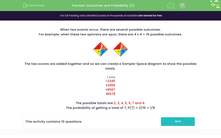# Use Sample Space Diagrams to Calculate Probability

In this worksheet, students will determine the possible outcomes of two events by using a sample space diagram.Key stage:  KS 3

Curriculum topic:   Probability

Curriculum subtopic:   Calculate Theoretical Probability

Difficulty level:#### Worksheet Overview

When two events occur, there are several possible outcomes.

For example, when these two spinners are spun, there are 4 x 4 = 16 possible outcomes.The two scores are added together and so we can create a sample space diagram to show the possible totals.

 1 2 3 4 1 2 3 4 5 2 3 4 5 6 3 4 5 6 7 4 5 6 7 8

The possible totals are 2, 3, 4, 5, 6, 7 and 8.

The probability of getting a total of 7, P(7) = 2/16 = 1/8